# Blackberries

Daniel, Jolana and Stano collected together 34 blackberries. Daniel collected 8 blackberries more than Jolana, Jolana 4 more than Stano. Determine the number blackberries each collected .

Correct result:

D =  18
J =  10
S =  6

#### Solution:

D+J+S=34
D = 8 + J
J = 4 + S

D+J+S = 34
D-J = 8
J-S = 4

D = 18
J = 10
S = 6

Our linear equations calculator calculates it.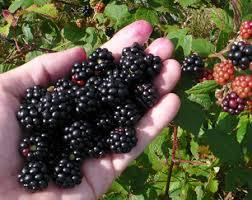We would be pleased if you find an error in the word problem, spelling mistakes, or inaccuracies and send it to us. Thank you!Tips to related online calculators
Do you have a linear equation or system of equations and looking for its solution? Or do you have quadratic equation?

## Next similar math problems:

• CoinsDenis and Zdeno together have 97 coins. If Denis had 4 coins less than he has now, the number of the coins would be in the ratio 14: 17. Determine the number of coins owned by Denis and Zdeno.
• PearsAndrew, Lenka and Rasto have together 232 pears. Lenka has 28 more than Rasto and Rasto pears have 96 more than Andrew. Determine how much each of them has pears.
• Quarantine cupcakes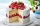Mr. Honse was baking quarantine cupcakes. Mrs. Carr made twice as many as Mr. Honse. Ms. Sanchez made 12 cupcakes more than Mr. Honse. If they put all their cupcakes together (which they can’t because. .. quarantine!) they would have 108 cupcakes. How may
• Sum of three numbersThe sum of three numbers from which second number is 20% smaller than the first number and the third number is 25% smaller than the second number is 96. Determine this numbers.
• Sugar - cuboid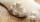Pejko received from his master cuboid composed of identical sugar cubes with count between 1000 and 2000. The Pejko eat sugar cubes in layers. The first day eat one layer from the front, second day one layer from right, the third day one layer above. Yet
• Three friends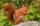Three friends squirrels together went to collect hazelnuts. Zrzecka he found more than twice Pizizubka and Ouska even three times more than Pizizubka. On the way home they talked while eating and was cracking her nuts. Pizizubka eaten half of all nuts whi
• Three friends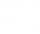Peter is eight times older than Rado, Rado is 3 times younger than Joseph. Together they have 120 years. Determine how many are each of them.
• EurosPeter, Jane and Thomas have together € 550. Tomas has 20 euros more than Jane, Peter € 150 less than Thomas. Determine how much has each of them.
• Iron collecting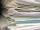Class 7A collected 3.2 tonnes of iron more than class 7B. Together they collected 6.4 tonnes of iron to the secondary raw material collection. How much did each class collect?
• HomeworkMother and Kate together weigh 24.5 kg more than the father. Father weighs 7.6 kg more than mother. Father weight 44.8 kg more than Kate. Determine weights of father mother and Kate.
• QuizQ2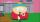The square of the first number is equal to three-fifths of the second number. Determine both numbers if you know that the second number is 5 times greater than the first, and neither of numbers is not equal to zero.
• Shoes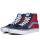Peter decided to measure shoes size of his three friends. Measured accurately and wrote as follows: Venda and Jenda together 52 cm Jenda and Zdenda together 58 cm Zdenda and Venda together 54 cm a) How long measures Vendy, Jenda and Zdenda shoes together?
• Water reservoir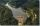The water reservoir is filled through one inlet 4 hours later than both together, then another inlet 9 hours later than both together. For how long is filled by each separately?
• MO Z8-I-1 2018Fero and David meet daily in the elevator. One morning they found that if they multiply their current age, they get 238. If they did the same after four years, this product would be 378. Determine the sum of the current ages of Fero and David.
• Stones 3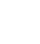Simiyu and Nasike each collected a number of stones in an arithmetic lesson. If Simiyu gave Nasike 5 stones, Nasike would have twice as many stones as Simiyu. If initially, Simiyu had five stones less than Nasike how many stones did each have?
• Three siblings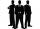Three siblings have birthday in one day-today. Together they have 35 years today. The youngest is three years younger than middle and the oldest is 5 years older than middle. How old is each?
• PotatoesDaniela and Michael would jointly dug potatoes for 7.5 hours. But if Daniela was working alone she would take 2.5 hours more as if he were working with Michael. Determine how much for the work done by Michael himself and how much Daniela herself.